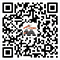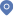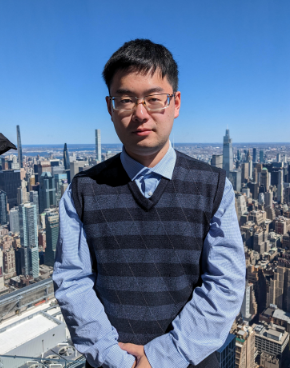Towards Provably Efficient Quantum Algorithms for Nonlinear Dynamics and Large-scale Machine Learning Models首页 - 学术活动

Dr. Jinpeng Liu, Simons Institute, UC Berkeley

Weiying Zheng, Professor

Towards Provably Efficient Quantum Algorithms for Nonlinear Dynamics and Large-scale Machine Learning Models

9:00-11:00 April 4 (Tuesday), Tencent Meeting: 509-892-294

Nonlinear dynamics play a prominent role in many domains and are notoriously difficult to solve. Whereas previous quantum algorithms for general nonlinear equations have been severely limited due to the linearity of quantum mechanics, we gave the first efficient quantum algorithm for nonlinear differential equations with sufficiently strong dissipation. This is an exponential improvement over the best previous quantum algorithms, whose complexity is exponential in the evolution time. We also established a lower bound showing that nonlinear differential equations with sufficiently weak dissipation have worst-case complexity exponential in time, giving an almost tight classification of the quantum complexity of simulating nonlinear dynamics. We applied the quantum algorithm for nonlinear reaction-diffusion equations for estimating thermodynamic free energies, laying the groundwork for practical applications of quantum computers for classical nonlinear systems.

Recently, we designed end-to-end quantum machine learning algorithms, combining efficient quantum (stochastic) gradient descent with sparse state preparation and sparse state tomography. We benchmarked instances of training sparse ResNet up to 103 million parameters, and identify the dissipative and sparse regime at the early phase of fine-tuning could receive quantum enhancement. Our work showed that fault-tolerant quantum algorithms could potentially contribute to the scalability and sustainability of most state-of-the-art, large-scale machine learning models.

References:

 Liu et al. Efficient quantum algorithm for dissipative nonlinear differential equations, Proceedings of the National Academy of Science 118, 35 (2021), arXiv:2011.03185.

 Liu et al. Towards provably efficient quantum algorithms for large-scale machine learning models, arXiv:2303.03428.

His research focuses on Quantum for Science. He attempts to develop, analyze, and optimize provably efficient quantum algorithms for computational challenges in natural and data sciences, including quantum simulations, quantum ODE/PDE solvers, q-sampling, and quantum gradient descent, toward end-to-end applications in areas such as quantum chemistry, biology and epidemiology, fluid dynamics, finance, statistics, optimization, and machine learning.